# Some pandas notes (part 1, EDA)

Teaching my son python at the moment – when I gave him a final project to do on his own, he complained and said he did not remember how to do one step from the prior lessons.

In the end, I am guessing I really only have maybe 30 commands in any language memorized, and then maybe another 70 I know of (but often need to look at the docs for its less often used arguments).

Here I figured it would be good for learners for me to put down my notes on pandas dataframes in python. At least the less than 20 commands I regularly use.

So first of I think a 3 part series, is using pandas for exploratory data analysis (EDA). Besides these tables I will show here, I often only use histograms and smooth conditional plots. Those I don’t even use very often.

Debated on writing a post that EDA is overrated, (I spend way more time understanding business objectives, then tuning a reasonably non-linear machine learning model and seeing its results dominates after that point). So it makes writing a post on EDA quite easy though – I can show a dozen pandas methods I regularly use and that is over 90% of the typical EDA work I do.

Here I will show an example using the PPP loan data available to download, in particular I will be using the over 150k loan data. A nice aspect of pandas is you can read in a CSV file directly from a url.

``````# Python code examples for EDA
import pandas as pd

# PPP loans, see full dataset
# at https://data.sba.gov/dataset/ppp-foia

# Can read in from url directly
pp_loans.shape # over 900k rows``````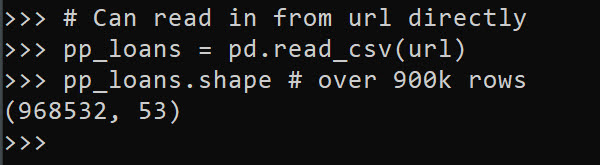At work I am often querying a database using SQLAlchemy instead of reading a csv file. But this is a nice example to show off – the data is too big to really work effectively in Excel, but pandas it should be no problem for most peoples machines.

So you can see the first thing I look at is `data.shape` to get the number of rows and columns. Next I often just print the columns using `list(data)`:

``````# Can use list to see all of the variables
list(pp_loans)``````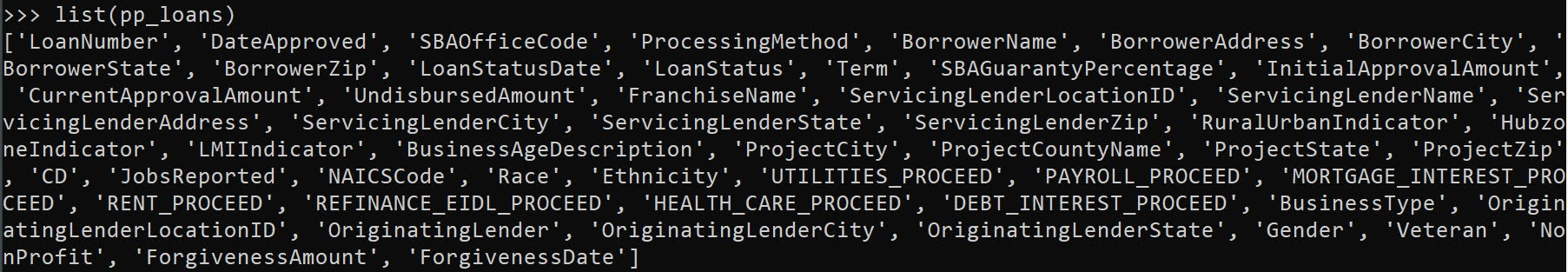So you can access/modify the actual column names via `data.columns`, but this is a bit nicer printing.

Next I often summarize the data using the `data.describe()` method. I like transposing this result to be easier to read though.

``````# Transpose describe for easier reading
pp_loans.describe().T``````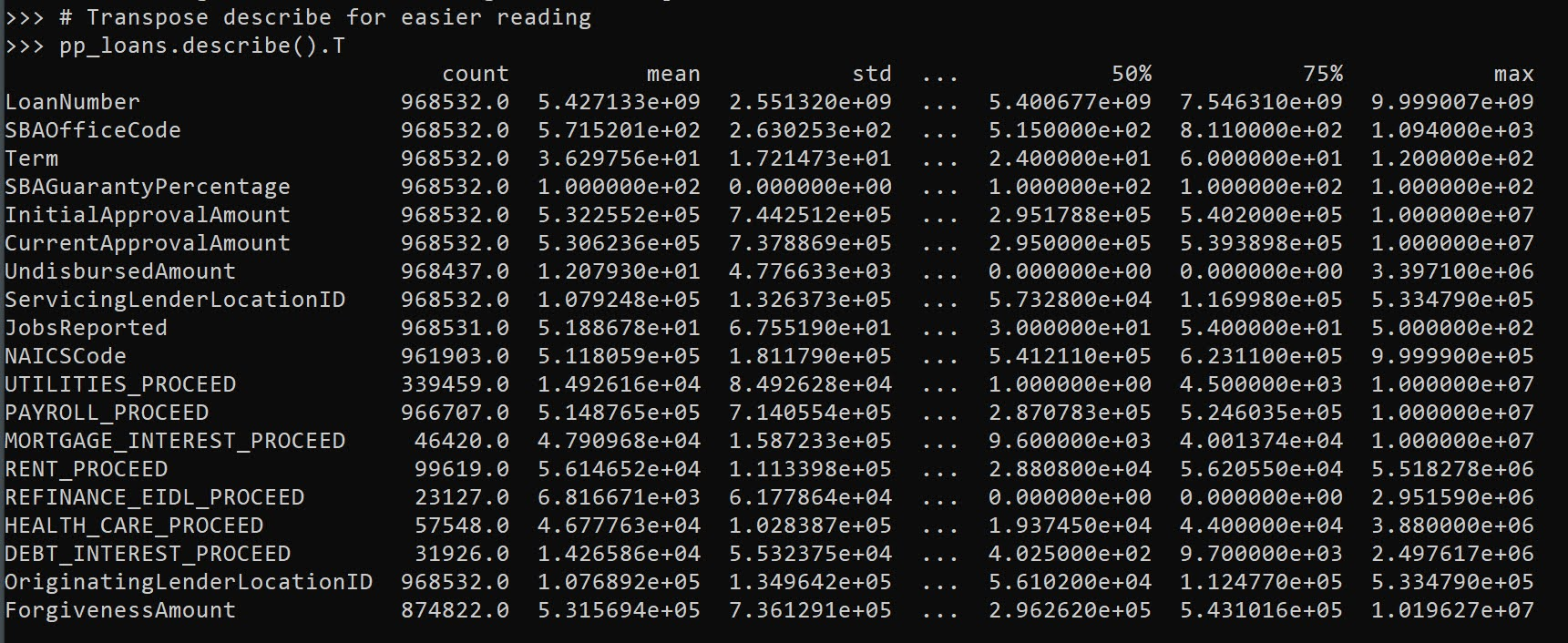Here we get a bit of a mess of scientific notation (also this only shows summaries of float data by default, not categorical or dates). If you want to set a bit easier on the eyes printing, I typically have this `pd.set_option()` handy. Also you can scale the data, here I divide everything by 1000.

``````# If you dont want scientific notation
pd.set_option('display.float_format', '{:,.0f}'.format)
pp_loans.describe().T/1000``````

One of the things I like about this the most is the count, so you can see data that has missing values (that here should likely be set to 0 for the `*_PROCEED` variables). The second thing I look at are the min/max. Sometimes you can spot rogue missing values or censoring in the data. Then looking at the quartiles is typically informative as to how skewed the data is. Only then do I bother with the mean – which for binary data is reasonably informative.

You can see for this particular data, it is the loans over \$150k – here this means apparently the `CurrentApprovalAmount`, as 150k to 10million (the apparent cap on the PPP loans). So this is \$150k conditional on approval dataset.

The next step I am typically looking the distribution of various categorical data. For that I am often just using `data['variable'].value_counts()`:

# Value counts is maybe my favorite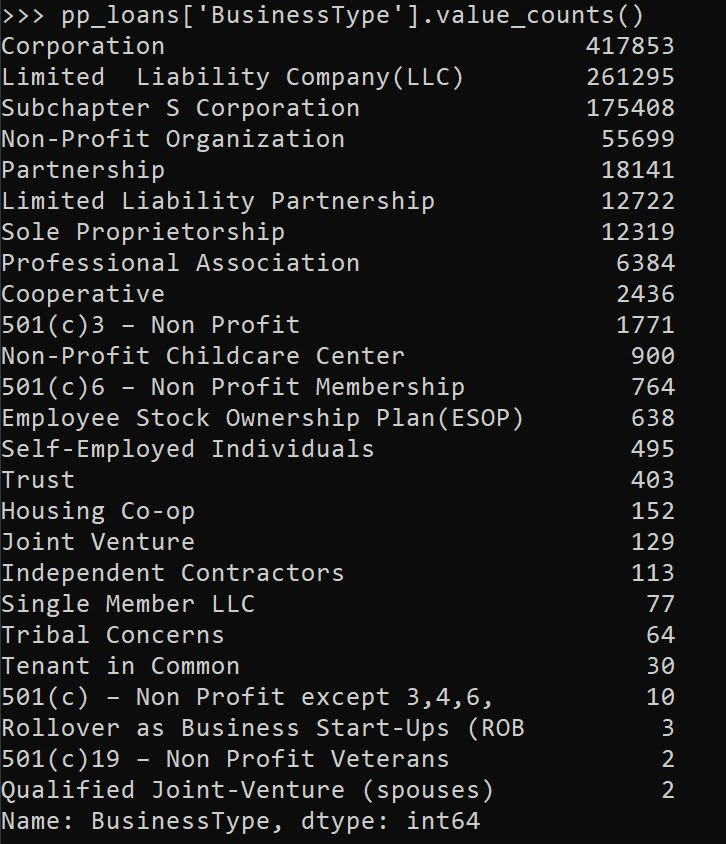This prints out all of the business types in this example, but with a very large number will limit to the head/tail. Here we can see Corps are the most common, with LLCs and s-corps as the next.

The next most common pandas method I use is `data.groupby()`, this produces summary aggregations conditional on different groups in the data. For example, here we can do the total sum of the loans (per million dollars) for each of the business types.

``````# Groupby with stats is next, per million
y = 'CurrentApprovalAmount'
pp_loans.groupby(x)[y].sum().sort_values()/1e6``````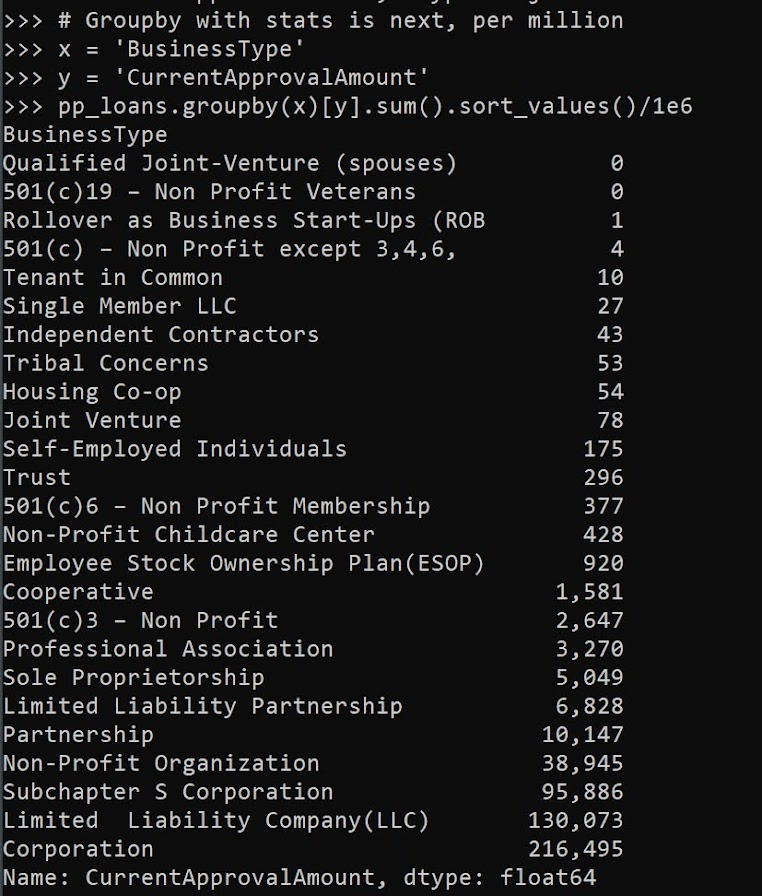You can see that you can chain multiple pandas methods together, which is just a typical python object oriented pattern. So I tack on a `.sort_values()` to the end of the groupby. Note again these are in millions of dollars, so Coop’s got over a billion dollars of PPP loans in this data (it will be more overall, these again are only loans over \$150k, the link at the beginning has a bunch of additional datasets with smaller loan amounts).

You can subsequently do additional functions in groupby, for just an example here I create my own normal distribution confidence interval of the mean for large samples.

``````# Can do more complicated functions
def ci95(x):
mx = x.mean()
sx = x.std()
res = pd.DataFrame([[mx - 2*sx,mx + 2*sx]])
res.columns = ['low','high']
return res

pp_loans.groupby(x)[y].apply(ci95)``````

I often use this for functions with binary data, but otherwise we can again chain methods together, so we can use `data.groupby(x)[y].describe()` to get those summaries for each of our groups. Also as an FYI you can pass in multiple `y` values to summarize via a list, although it gets a bit hairy in the output:

``````# And can have multiple outcome variables
ys = [y,'PAYROLL_PROCEED']
pp_loans.groupby(x)[ys].describe()``````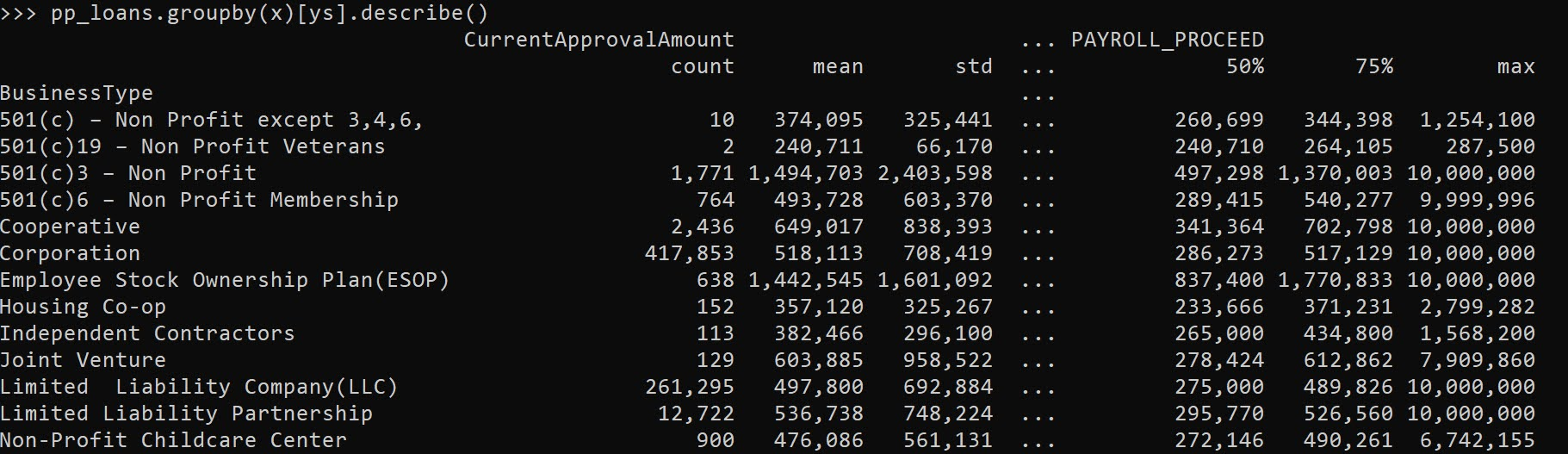One of the common things I do at this point is to simply dump the results to a CSV file I can lookat, e.g. something like:

``````# Can chain together and save result to csv
pp_loans.groupby(x)[ys].describe().to_csv('aggstats.csv')``````

Or assign to a new object, and some python editors (such as Spyder) have a data browsers.

The final EDA part I use most often is simply sorting the dataset and looking at the head/tail.

``````# Can sort values and look at head/tail
pp_loans.sort_values(by=ys,ascending=False,inplace=True,ignore_index=True)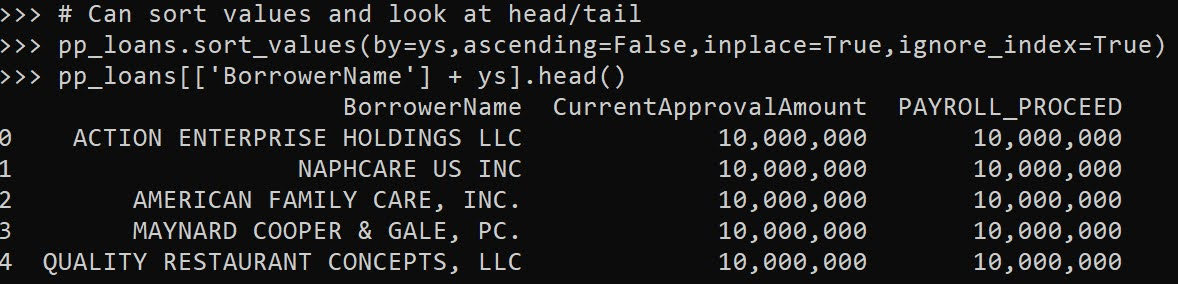I often will export the head of the data, e.g. something like `data.head().to_csv('checkdata.csv')` to view all of the fields and their content as well when first figuring out fields, how often they are filled in, etc.

Next part in the series, I will describe more regular pandas data manipulation operations I regularly use, e.g. getting a single column vs multiple columns, filtering, string manipulation functions, etc.

# Chunking it up in pandas

In the python pandas library, you can read a table (or a query) from a SQL database like this:

``data = pandas.read_sql_table('tablename',db_connection)``

Pandas also has an inbuilt function to return an iterator of chunks of the dataset, instead of the whole dataframe.

``data_chunks = pandas.read_sql_table('tablename',db_connection,chunksize=2000)``

I thought for awhile this was somewhat worthless, as I thought it still read the whole thing into memory. I have been using it though to pull new records, generate predictions, and then feed the predictions back into another table though (easier to write back to a new DB in smaller chunks).

But I actually did some tests recently and I was wrong. When using `chunksize`, it does not read the whole table into memory, so works like you would want. In particular, I was testing out sqlalchemy and `execution_options=dict(stream_results=True)` when creating the engine. Which works great for postgres – it is not needed though for every other DB I have tried so far (I will show postgres and sqlite here, at work have also tested teradata and sql server).

Here I use the python library memory_profiler and show the difference in memory usage for a database of crime incidents from Dallas PD. First in my script I load in the libraries I will be using, and then created my different engine connection strings

``````'''
Tests for memory and chunking
up dataframes
'''
import sqlalchemy
import pandas as pd
from datetime import datetime
from memory_profiler import profile

# Check out https://github.com/apwheele/Blog_Code/tree/master/Python/jupyter_reports
# For where this data came from
# sqlalchemy string for SQLlite
sqli = r'sqlite:///D:\Dropbox\Dropbox\PublicCode_Git\Blog_Code\Python\jupyter_reports\DPD.sqlite'
lite_eng = sqlalchemy.create_engine(sqli)

# sqlalchemy string for postgres
pg = f'postgresql://id:pwd@localhost/dbname' #need to fill these in with your own info
pg_eng = sqlalchemy.create_engine(pg)
pg_eng_str = sqlalchemy.create_engine(pg, execution_options=dict(stream_results=True))``````

Next, we can make a function to either read a table all at once, or to return an iterator that chunks the data up into a tinier number of rows. We also decorate this function with the profile function to get some nice statistics later. Here I just do some random stats and accumulate them in each iteration.

``````@profile
begin_time = datetime.now()
# If chunk, do the calcs in iterations
if chnk:
tot_inc, tot_arr = 0,0
for c in inc:
tot_inc += c.shape
tot_arr += (c['Offense_Status'] == 'Clear by Arrest').sum()
else:
tot_inc = inc.shape
tot_arr = (inc['Offense_Status'] == 'Clear by Arrest').sum()
print(f'\nsql: {sql_con}\nTotal incidents {tot_inc}\nTotal arrests {tot_arr}\nRuntime {begin_time} -- {datetime.now()}\n')``````

Most of the time I am using something like this for predictions or generating follow up metrics to see how well my model is doing (and rewriting those intermediate results to a table). So inside the iteration loop looks something like `pred_val = predict_function(c)` and `pred_val.to_sql('new_table',sql_con,if_exists='append')`.

Now just to finish off the script, you can do run the tests if called directly:

``````if __name__ == "__main__":
read_table(sqli,None)       #sqlite big table all at once
read_table(sqli,2000)       #sqlite in chunks
read_table(pg_eng,None)     #pg big table
read_table(pg_eng,2000)     #pg in chunks
read_table(pg_eng_str,2000) #pg stream in chunks``````

Now when running this script, you would run it as `python -m memory_profiler chunk_tests.py > test_results.txt`, and this will give you a nice set of print outs for each function call. So lets start with sqlite reading the entire database into memory:

``````sql: sqlite:///D:\Dropbox\Dropbox\PublicCode_Git\Blog_Code\Python\jupyter_reports\DPD.sqlite
Total incidents 785064
Total arrests 89741
Runtime 2021-08-11 19:22:41.652316 -- 2021-08-11 19:23:53.743702

Filename: chunk_tests.py

Line #    Mem usage    Increment  Occurences   Line Contents
============================================================
28     82.4 MiB     82.4 MiB           1   @profile
29                                         def read_table(sql_con, chnk=None):
30     82.4 MiB      0.0 MiB           1       begin_time = datetime.now()
31   4260.7 MiB   4178.3 MiB           1       inc = pd.read_sql_table('incidents',sql_con,chunksize=chnk)
32                                             # If chunk, do the calcs in iterations
33   4260.7 MiB      0.0 MiB           1       if chnk:
34                                                 tot_inc, tot_arr = 0,0
35                                                 for c in inc:
36                                                     tot_inc += c.shape
37                                                     tot_arr += (c['Offense_Status'] == 'Clear by Arrest').sum()
38                                             else:
39   4260.7 MiB      0.0 MiB           1           tot_inc = inc.shape
40   4261.5 MiB      0.8 MiB           1           tot_arr = (inc['Offense_Status'] == 'Clear by Arrest').sum()
41   4261.5 MiB      0.0 MiB           1       print(f'\nsql: {sql_con}\nTotal incidents {tot_inc}\nTotal arrests {tot_arr}\nRuntime {begin_time} -- {datetime.now()}\n')``````

You can see that the incident database is 785k rows (it is 100 columns as well). It takes only alittle over a minute to read in the table, but it takes up over 4 gigabytes of memory. So I can fit this in memory on my machine, but if I say added a free text comments field I might not be able to fit this in memory on my personal machine.

Now lets see what these results look like when I chunk the data up into smaller sets of only 2000 rows at a time:

``````sql: sqlite:///D:\Dropbox\Dropbox\PublicCode_Git\Blog_Code\Python\jupyter_reports\DPD.sqlite
Total incidents 785064
Total arrests 89741
Runtime 2021-08-11 19:23:56.475680 -- 2021-08-11 19:25:33.896884

Filename: chunk_tests.py

Line #    Mem usage    Increment  Occurences   Line Contents
============================================================
28     91.6 MiB     91.6 MiB           1   @profile
29                                         def read_table(sql_con, chnk=None):
30     91.6 MiB      0.0 MiB           1       begin_time = datetime.now()
31     91.9 MiB      0.3 MiB           1       inc = pd.read_sql_table('incidents',sql_con,chunksize=chnk)
32                                             # If chunk, do the calcs in iterations
33     91.9 MiB      0.0 MiB           1       if chnk:
34     91.9 MiB      0.0 MiB           1           tot_inc, tot_arr = 0,0
35    117.3 MiB    -69.6 MiB         394           for c in inc:
36    117.3 MiB    -89.6 MiB         393               tot_inc += c.shape
37    117.3 MiB    -89.6 MiB         393               tot_arr += (c['Offense_Status'] == 'Clear by Arrest').sum()
38                                             else:
39                                                 tot_inc = inc.shape
40                                                 tot_arr = (inc['Offense_Status'] == 'Clear by Arrest').sum()
41    112.0 MiB     -5.3 MiB           1       print(f'\nsql: {sql_con}\nTotal incidents {tot_inc}\nTotal arrests {tot_arr}\nRuntime {begin_time} -- {datetime.now()}\n')``````

We only end up maxing out the memory at under 120 megabytes. You can see the total function also only takes around 1.5 minutes. So it is only ~30 seconds longer to run this result in chunks than doing it all at once.

Now onto postgres, I will forego showing the results when reading in the whole table at once – it is pretty much the same as sqlite when reading the whole table at once. But here are the results for the chunks with the usual sqlalchemy connection string:

``````sql: Engine(postgresql://postgres:***@localhost/postgres)
Total incidents 785064
Total arrests 89741
Runtime 2021-08-11 19:26:28.454807 -- 2021-08-11 19:27:41.917612

Filename: chunk_tests.py

Line #    Mem usage    Increment  Occurences   Line Contents
============================================================
28     51.3 MiB     51.3 MiB           1   @profile
29                                         def read_table(sql_con, chnk=None):
30     51.3 MiB      0.0 MiB           1       begin_time = datetime.now()
31   2017.2 MiB   1966.0 MiB           1       inc = pd.read_sql_table('incidents',sql_con,chunksize=chnk)
32                                             # If chunk, do the calcs in iterations
33   2017.2 MiB      0.0 MiB           1       if chnk:
34   2017.2 MiB      0.0 MiB           1           tot_inc, tot_arr = 0,0
35   2056.8 MiB  -1927.3 MiB         394           for c in inc:
36   2056.8 MiB      0.0 MiB         393               tot_inc += c.shape
37   2056.8 MiB      0.0 MiB         393               tot_arr += (c['Offense_Status'] == 'Clear by Arrest').sum()
38                                             else:
39                                                 tot_inc = inc.shape
40                                                 tot_arr = (inc['Offense_Status'] == 'Clear by Arrest').sum()
41     89.9 MiB  -1966.9 MiB           1       print(f'\nsql: {sql_con}\nTotal incidents {tot_inc}\nTotal arrests {tot_arr}\nRuntime {begin_time} -- {datetime.now()}\n')``````

So here we get some savings, but not nearly as good as sqlite – it ends up reading in around 2 gig for the iterator. But no fear, there is a specific option when setting up the sqlalchemy string for postgres. If you pay close attention to above where I build the connection strings, the engine for this trial is `pg_eng_str = sqlalchemy.create_engine(pg, execution_options=dict(stream_results=True))`. And when I use the streaming results engine, here is the memory profile I get:

``````sql: Engine(postgresql://postgres:***@localhost/postgres)
Total incidents 785064
Total arrests 89741
Runtime 2021-08-11 19:27:41.922613 -- 2021-08-11 19:28:54.435834

Filename: chunk_tests.py

Line #    Mem usage    Increment  Occurences   Line Contents
============================================================
28     88.8 MiB     88.8 MiB           1   @profile
29                                         def read_table(sql_con, chnk=None):
30     88.8 MiB      0.0 MiB           1       begin_time = datetime.now()
31     89.6 MiB      0.8 MiB           1       inc = pd.read_sql_table('incidents',sql_con,chunksize=chnk)
32                                             # If chunk, do the calcs in iterations
33     89.6 MiB      0.0 MiB           1       if chnk:
34     89.6 MiB      0.0 MiB           1           tot_inc, tot_arr = 0,0
35    100.5 MiB   -322.6 MiB         394           for c in inc:
36    100.5 MiB   -325.4 MiB         393               tot_inc += c.shape
37    100.5 MiB   -325.4 MiB         393               tot_arr += (c['Offense_Status'] == 'Clear by Arrest').sum()
38                                             else:
39                                                 tot_inc = inc.shape
40                                                 tot_arr = (inc['Offense_Status'] == 'Clear by Arrest').sum()
41     92.3 MiB     -8.2 MiB           1       print(f'\nsql: {sql_con}\nTotal incidents {tot_inc}\nTotal arrests {tot_arr}\nRuntime {begin_time} -- {datetime.now()}\n')``````

Which is very similar to sqlite results.

Some workflows unfortunately are not so reducable into tiny chunks like this. E.g. say you were doing multiple time series for different groups, you would likely need to use substitution into the SQL to get one group at a time. I have done this to grab chunks of claims data one month at a time, but in many of my workflows I could just replace that with chunksize and do one read.

Some folks reading this may be thinking “this is a great use case for hadoop”. For the most part I am personally not working with that big of data, just mildly annoying at the edge of fitting into memory data. So this just do stuff in chunks works great for most of my workflows, and I don’t have to deal with the hassles of hadoop/spark.

# Histogram notes in python with pandas and matplotlib

Here are some notes (for myself!) about how to format histograms in python using pandas and matplotlib. The defaults are no doubt ugly, but here are some pointers to simple changes to formatting to make them more presentation ready.

First, here are the libraries I am going to be using.

``````import pandas as pd
import numpy as np
import matplotlib
from matplotlib import pyplot as plt
from matplotlib.ticker import StrMethodFormatter
from matplotlib.ticker import FuncFormatter``````

Then I create some fake log-normal data and three groups of unequal size.

``````#generate three groups of differing size
n = 50000
group = pd.Series(np.random.choice(3, n, p=[0.6, 0.35, 0.05]))
#generate log-normal data
vals = pd.Series(np.random.lognormal(mean=(group+2)*2,sigma=1))
dat = pd.concat([group,vals], axis=1)
dat.columns = ['group','vals']``````

And note I change my default plot style as well. So if you are following along your plots may look slightly different than mine.

One trick I like is using `groupby` and describe to do a simple textual summary of groups. But I also like transposing that summary to make it a bit nicer to print out in long format. (I use spyder more frequently than notebooks, so it often cuts off the output.) I also show setting the pandas options to a print format with no decimals.

``````#Using describe per group
pd.set_option('display.float_format', '{:,.0f}'.format)
print( dat.groupby('group')['vals'].describe().T )``````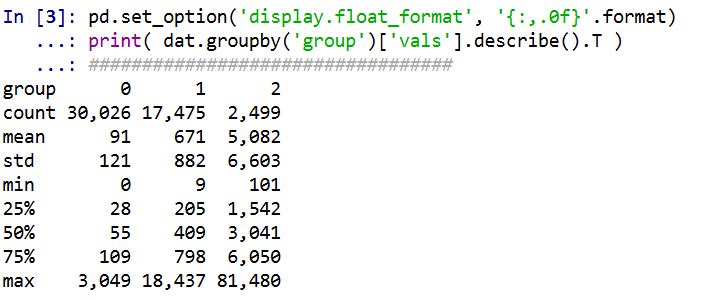Now onto histograms. Pandas has many convenience functions for plotting, and I typically do my histograms by simply upping the default number of bins.

``dat['vals'].hist(bins=100, alpha=0.8)``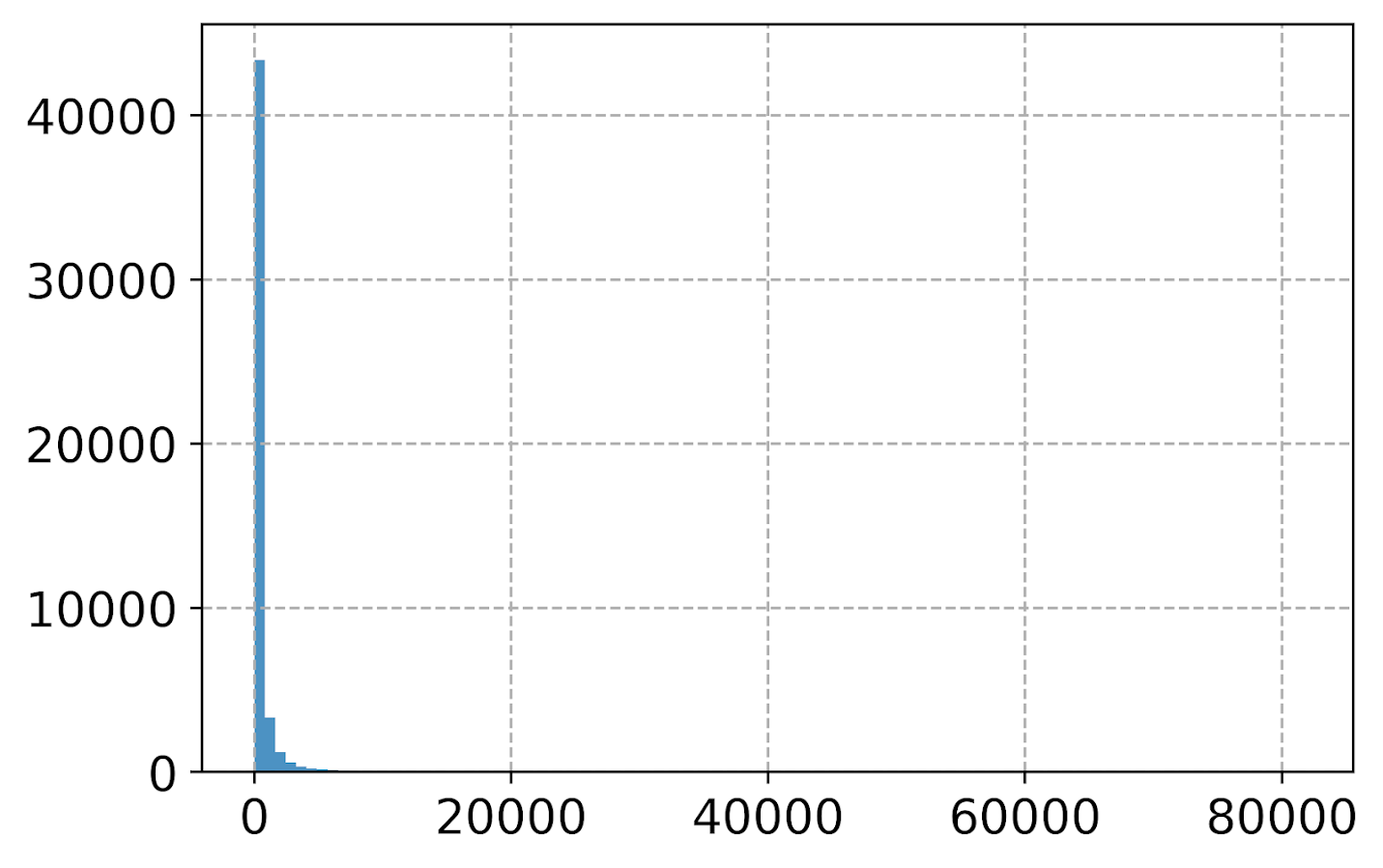Well that is not helpful! So typically when I see this I do a log transform. (Although note if you are working with low count data that can have zeroes, a square root transformation may make more sense. If you have only a handful of zeroes you may just want to do something like `np.log([dat['x'].clip(1))` just to make a plot on the log scale, or some other negative value to make those zeroes stand out.)

``````#Histogram On the log scale
dat['log_vals'] = np.log(dat['vals'])
dat['log_vals'].hist(bins=100, alpha=0.8)``````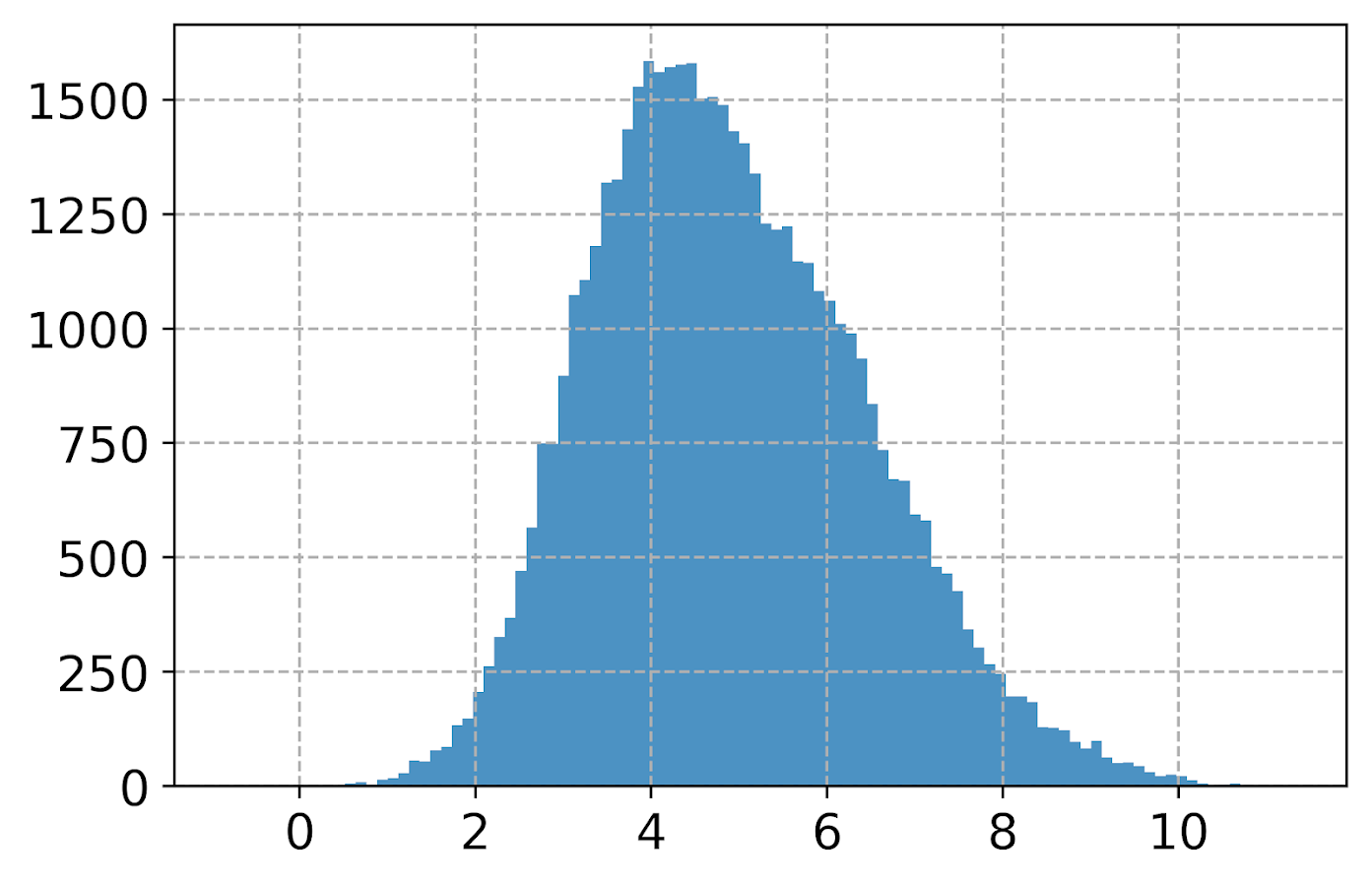Much better! It may not be obvious, but using pandas convenience plotting functions is very similar to just calling things like `ax.plot` or `plt.scatter` etc. So you can assign the plot to an axes object, and then do subsequent manipulations. (Don’t ask me when you should be putzing with axes objects vs plt objects, I’m just muddling my way through.)

So here is an example of adding in an X label and title.

``````#Can add in all the usual goodies
ax = dat['log_vals'].hist(bins=100, alpha=0.8)
plt.title('Histogram on Log Scale')
ax.set_xlabel('Logged Values')``````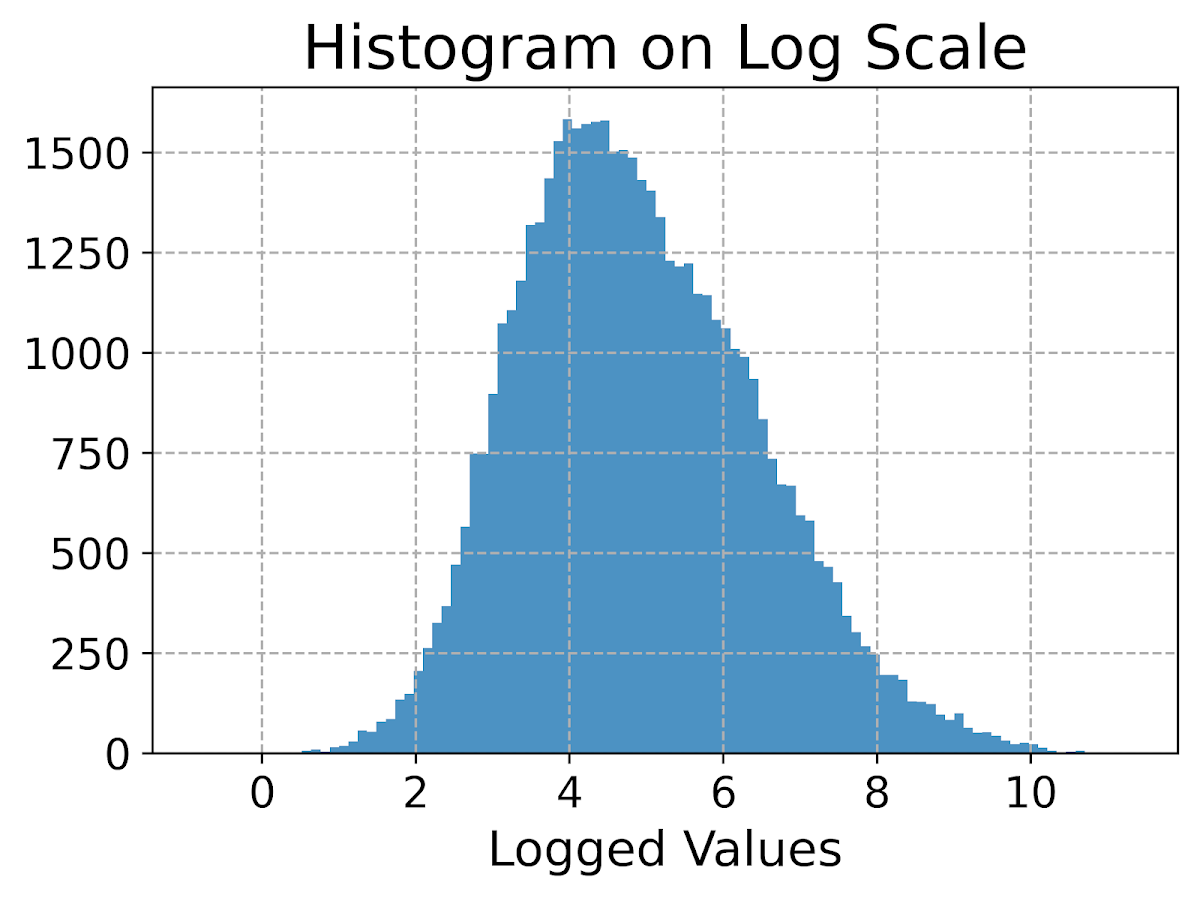Although it is hard to tell in this plot, the data are actually a mixture of three different log-normal distributions. One way to compare the distributions of different groups are by using `groupby` before the histogram call.

``````#Using groupby to superimpose histograms
dat.groupby('group')['log_vals'].hist(bins=100)``````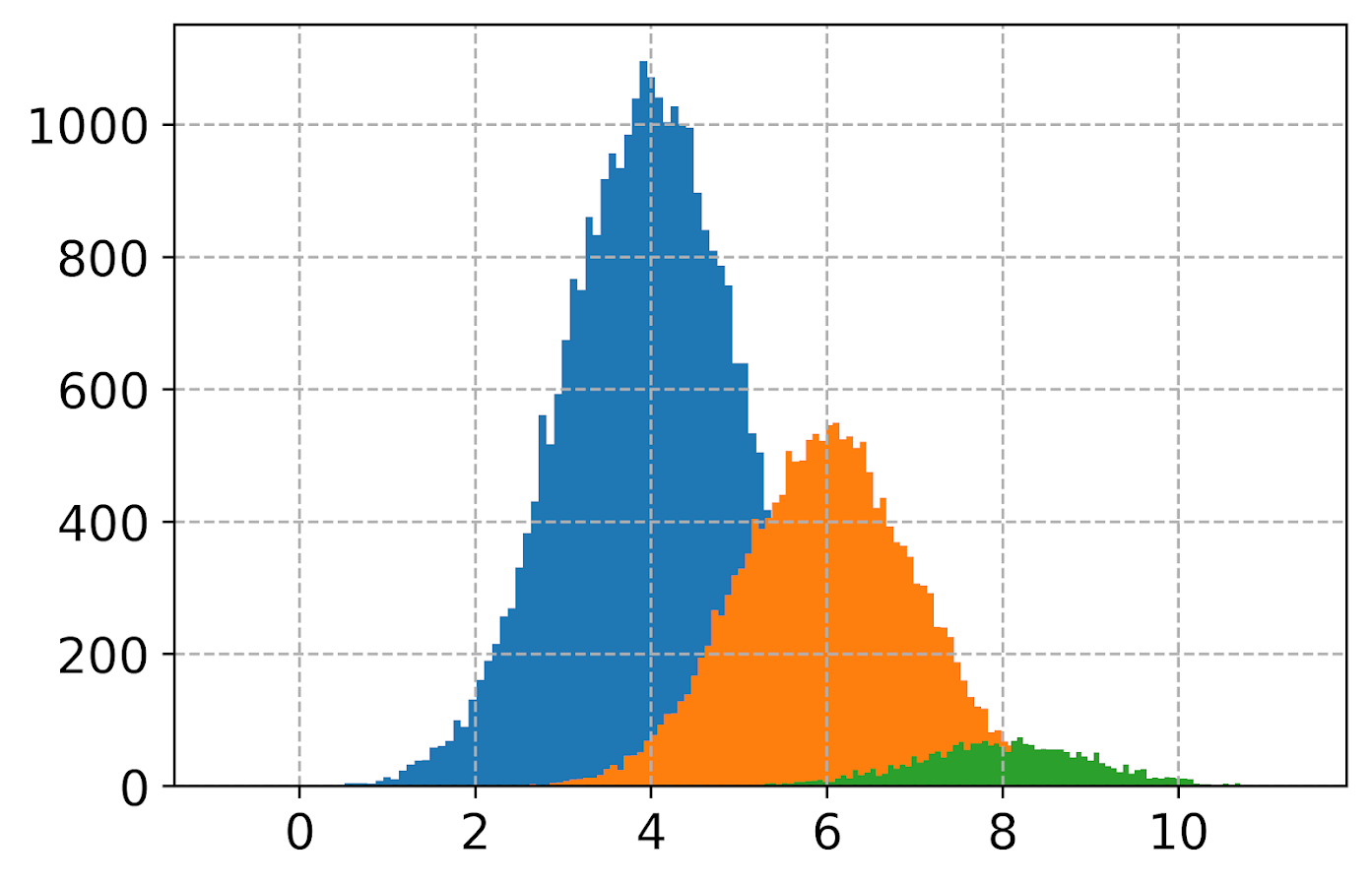But you see here two problems, since the groups are not near the same size, some are shrunk in the plot. The second is I don’t know which group is which. To normalize the areas for each subgroup, specifying the `density` option is one solution. Also plotting at a higher alpha level lets you see the overlaps a bit more clearly.

``dat.groupby('group')['log_vals'].hist(bins=100, alpha=0.65, density=True)``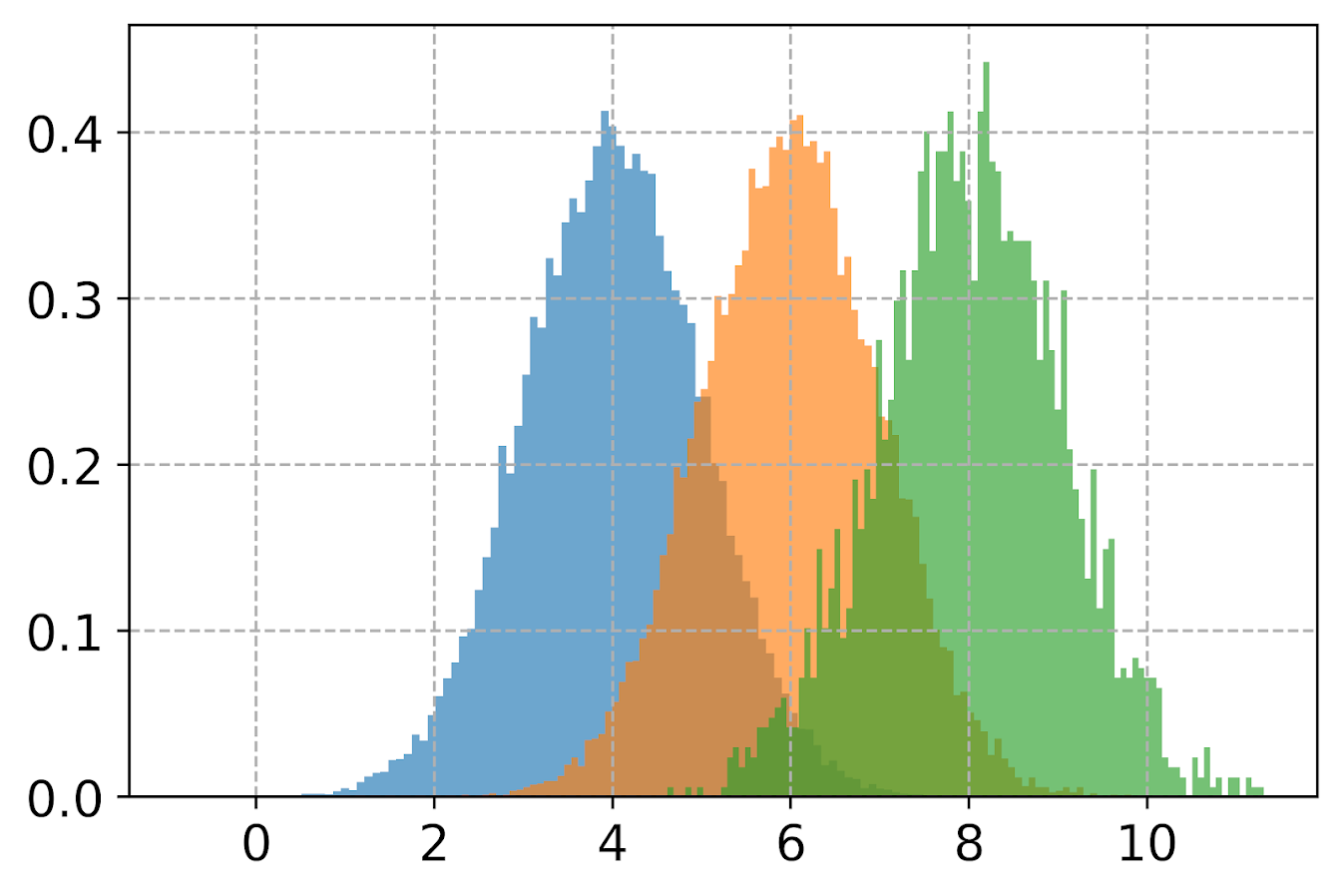Unfortunately I keep getting an error when I specify `legend=True` within the `hist()` function, and specifying `plt.legend` after the call just results in an empty legend. So another option is to do a small multiple plot, by specifying a `by` option within the `hist` function (instead of `groupby`).

``````#Small multiple plot
dat['log_vals'].hist(bins=100, by=dat['group'],
alpha=0.8, figsize=(8,8))``````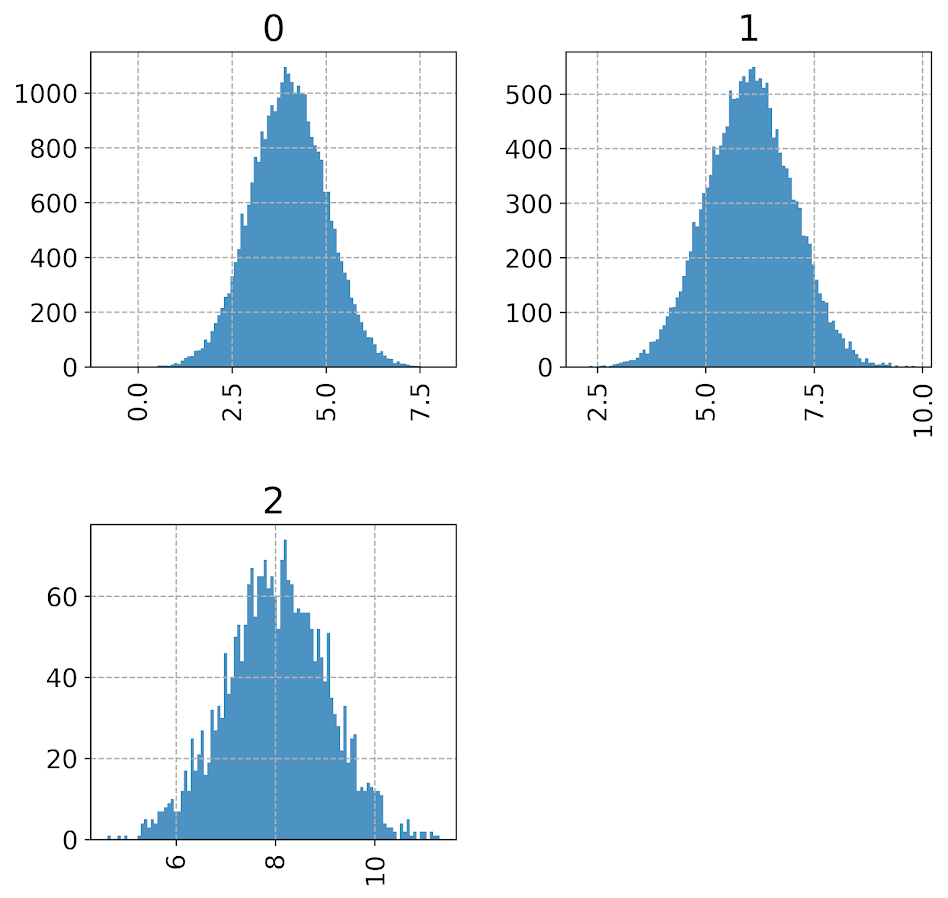This takes up more room, so can pass in the `figsize()` parameter directly to expand the area of the plot. Be careful when interpreting these, as all the axes are by default not shared, so both the Y and X axes are different, making it harder to compare offhand.

Going back to the superimposed histograms, to get the legend to work correctly this is the best solution I have come up with, just simply creating different charts in a loop based on the subset of data. (I think that is easier than building the legend yourself.)

``````#Getting the legend to work!
for g in pd.unique(dat['group']):
dat.loc[dat['group']==g,'log_vals'].hist(bins=100,alpha=0.65,
label=g,density=True)
plt.legend(loc='upper left')``````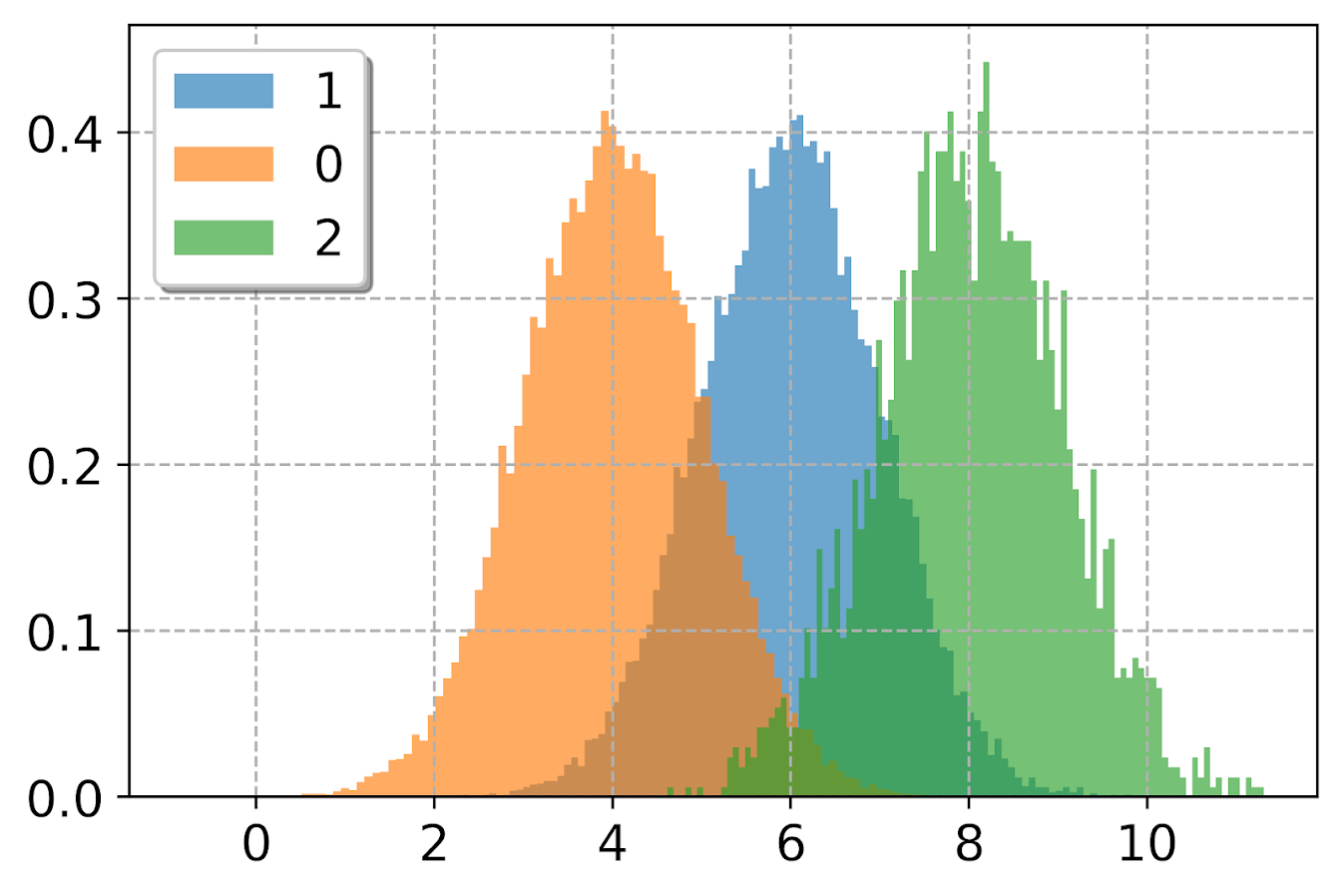Besides the `density=True` to get the areas to be the same size, another trick that can sometimes be helpful is to weight the statistics by the inverse of the group size. The Y axis is not really meaningful here, but this sometimes is useful for other chart stats as well.

``````#another trick, inverse weighting
dat['inv_weights'] = 1/dat.groupby('group')['vals'].transform('count')
for g in pd.unique(dat['group']):
sub_dat = dat[dat['group']==g]
sub_dat['log_vals'].hist(bins=100,alpha=0.65,
label=g,weights=sub_dat['inv_weights'])
plt.legend(loc='upper left')    ``````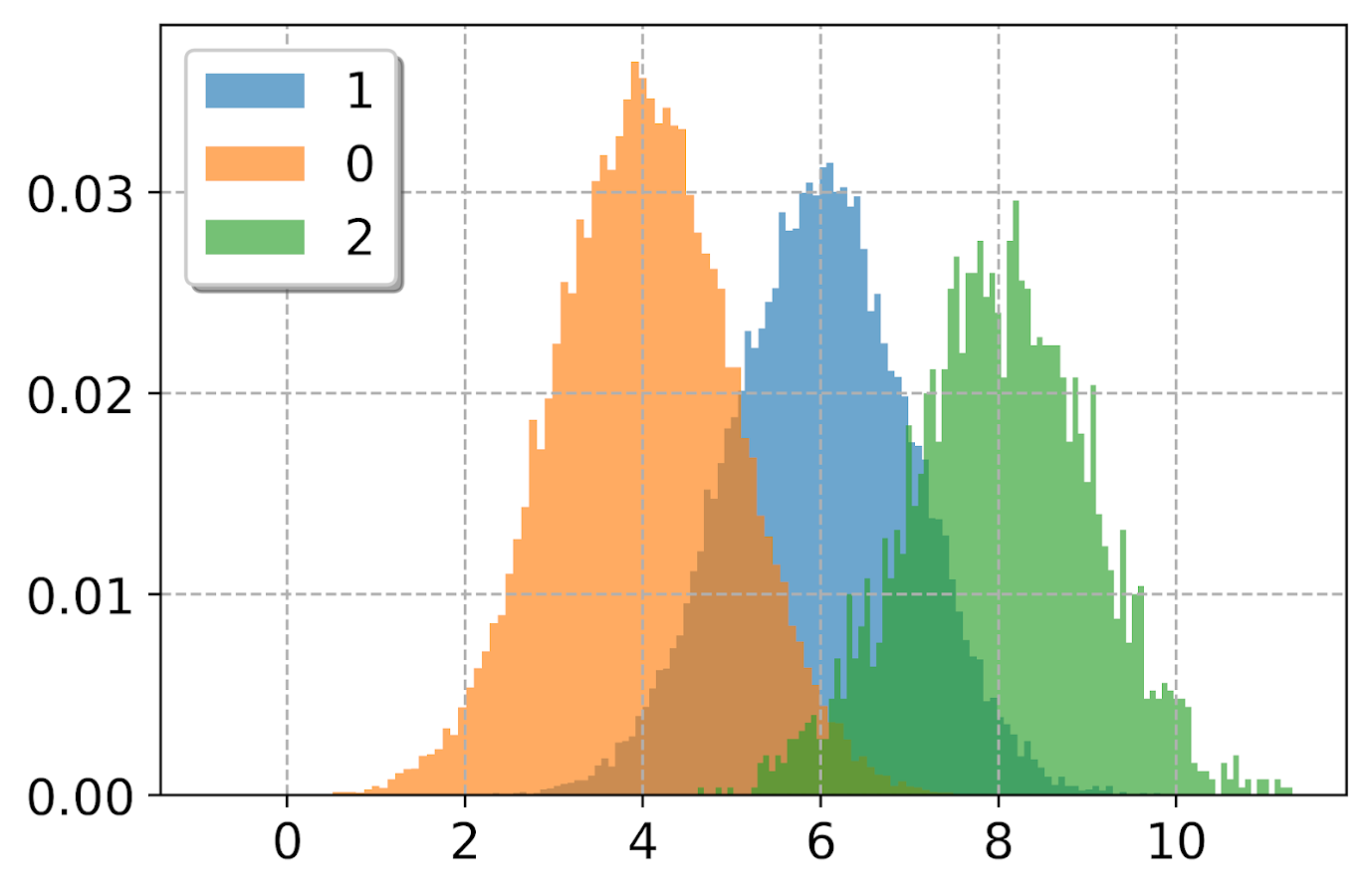So far, I have plotted the logged values. But I often want the labels to show the original values, not the logged ones. There are two different ways to deal with that. One is to plot the original values, but then use a log scale axis. When you do it this way, you want to specify your own bins for the histogram. Here I also show how you can use `StrMethodFormatter` to return a money value. Also rotate the labels so they do not collide.

``````#Specifying your own bins on original scale
#And using log formatting
log_bins = np.exp(np.arange(0,12.1,0.1))
ax = dat['vals'].hist(bins=log_bins, alpha=0.8)
plt.xscale('log', basex=10)
ax.xaxis.set_major_formatter(StrMethodFormatter('\${x:,.0f}'))
plt.xticks(rotation=45)``````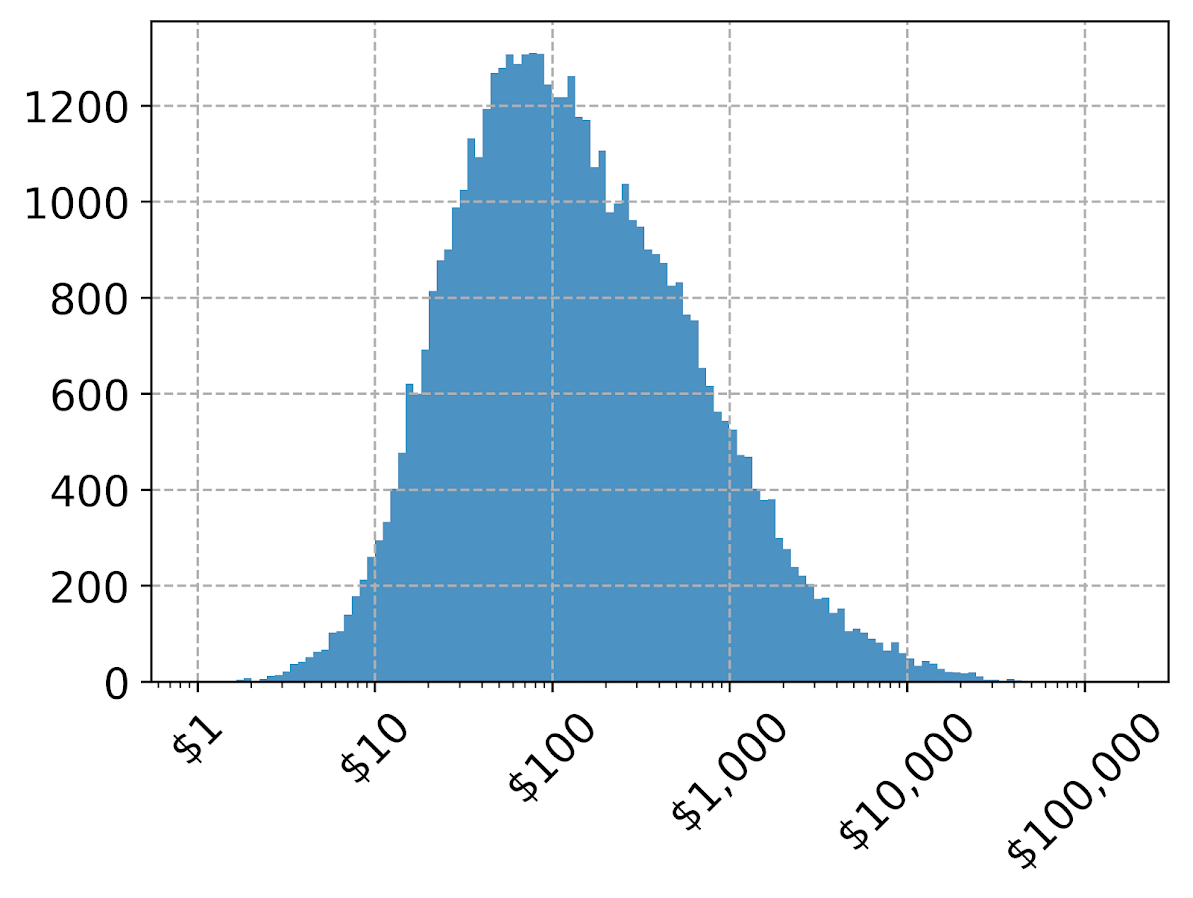If you omit the formatter option, you can see the returned values are `10^2`, `10^3` etc. Besides log base 10, folks should often give log base 2 or log base 5 a shot for your data.

Another way though is to use our original logged values, and change the format in the chart. Here we can do that using `FuncFormatter`.

``````#Using the logged scaled, then a formatter
#https://napsterinblue.github.io/notes/python/viz/tick_string_formatting/
def exp_fmt(x,pos):
return '\${:,.0f}'.format(np.exp(x))
fmtr = FuncFormatter(exp_fmt)

ax = dat['log_vals'].hist(bins=100, alpha=0.8)
plt.xticks(np.log([5**i for i in range(7)]))
ax.xaxis.set_major_formatter(fmtr)
plt.xticks(rotation=45)``````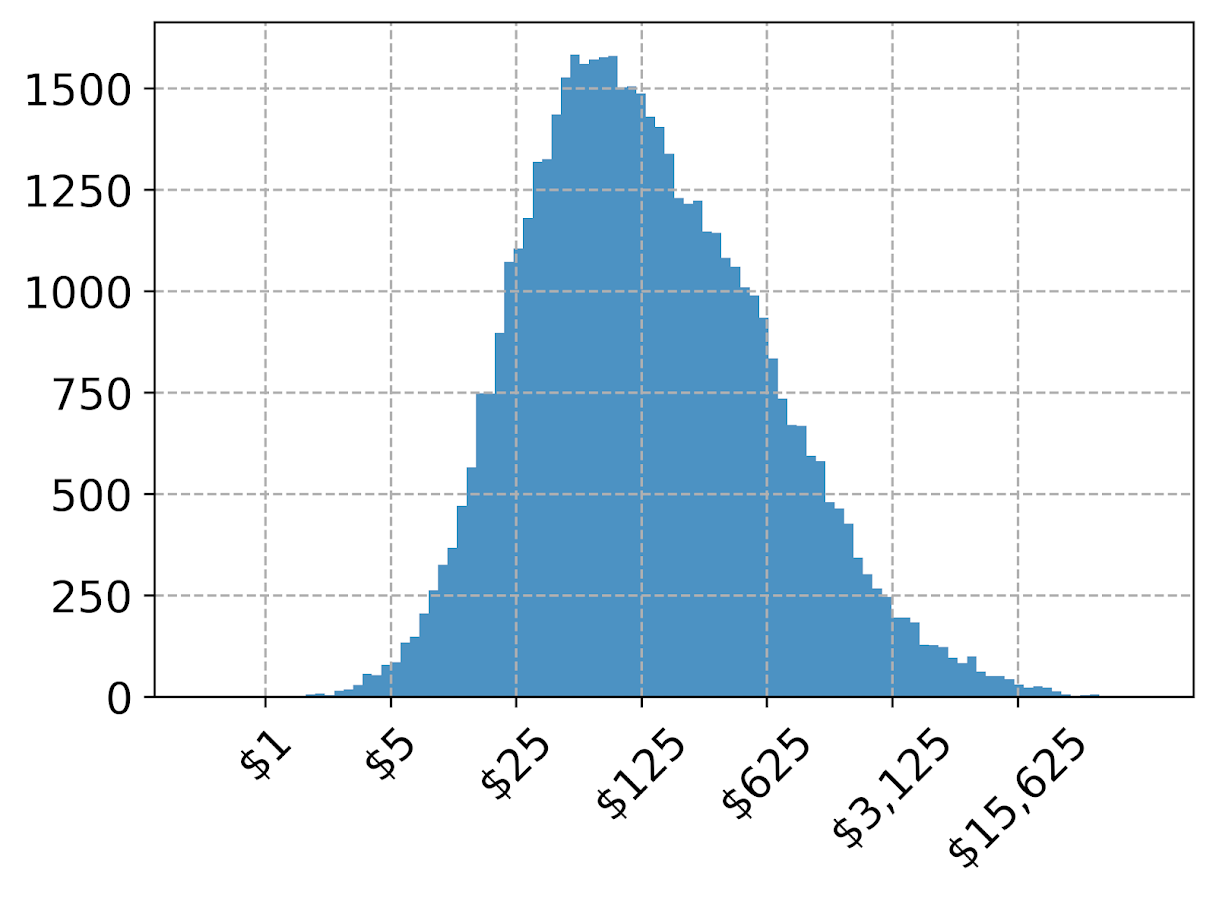On the slate is to do some other helpers for scatterplots and boxplots. The panda defaults are no doubt good for EDA, but need some TLC to make more presentation ready.